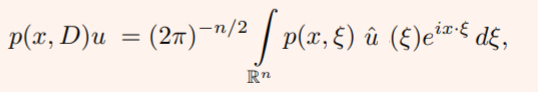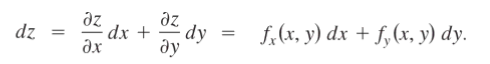# Differential Operator, Pseudodifferential, Total & Forms

## 1. Differential Operator

A differential operator tells you to differentiate (take the derivative) with respect to some variable. Typically, the variable differentiated with respect to is x.

Algebraically, the differential operator with respect to x is written as:

d/dx or Dx

The differential operator can also be applied to other variables provided they are a function of x. For example, when y is a function of x, it’s written as:

d/dx(y) = dy/dx

When working with differential operators you use the same rules of differentiation to find the derivative of a function. The simplest differential operator action on a function y gives us the first derivative of the function:

Dy(x) = y′(x)

You can also get the second derivative of the function y(x):

D2y(x) = D(Dy(x)) = Dy′(x) = y′′(x)

## Examples: Differential Operator

A typical question will ask you to apply the differential operator to an equation or expression.

Example 1: Apply the differential operator to the expression 5x4

The answer will be the derivative of 5xwith respect to x. To get the first derivative, just differentiate once, in this case applying the Power Rule:

d/dx (5x4) = 20x4

When you apply the operator to expression 5x4 the answer is 20x4.

Questions may also ask you to apply the differential operator multiple times. These questions are asking you to find higher derivatives.

Example 2: Apply the differential operator twice to y = 5x4

In the first example, applying the operator once resulted in dy/dx = 20x3. To apply it a second time we write:

d/dx (dy/dx) = d/dx (20x3)

The left-hand side of the equation becomes:

d2y/dx2

Apply the Power Rule again to the right-hand side:

d/dx (20x3) = 60x2

Finally, combine the two sides to get the second derivative of y = 5x2

d2y/dx2 = 60x2

## 2. Pseudodifferential Operator

Pseudodifferential Operators are a generalization of differential operators. This generalization means that every differential operator is a pseudodifferential operator; In other words, pseudodifferentials are obtained by enlarging the class of differential operators. They originated in the latter half of the 20th century at the beginning of elliptic theory, as a natural class of operators which contained the near-inverses of elliptic differential operators.

The inverse of a pseudodifferential operator is also a pseudodifferential operator (Nagy, 2004).

## Pseudodifferential Operators Uses

Pseudodifferential operators have a wide variety of applications. They are frequently used:

• In the theory of partial differential equations (PDEs), where many natural objects are pseudodifferentials including the inverse of an elliptic operator,
• In elliptic theory, as a tool on smooth manifolds. They also arise naturally in the classification of elliptic operators and in the reduction of elliptic boundary problems to the boundary,
• Boundary value problems for hyperbolic equations (Treves, 1980),
• Many other practical and theoretical problems in quantum field theory and signal processing.

## Representation of Pseudo Differential Operators

The Fourier representation of the operator, represented by p(x, ξ), is:You can think of a differential operator as something that acts on a function as the inverse Fourier transform of a polynomial in the Fourier variable multiplied by the Fourier transform of the function.

Another representation is as a singular integral (Taylor, 2008):

p(x, D)u = ∬ K (x, x y) u (y) dy,
Where K (x, x y) = (2π)-np (x, Ξ) ei(x – y) · Ξ

## Weyl and Kohn-Nirenberg correspondences

While there are many ways to construct pseudodifferential operators, Weyl and Kohn-Nirenberg correspondences are two of the most popular. In quantum mechanics, these are both examples of “quantization rules” (Heil, 2003).

As pseudodifferential operators span an immense field of work, it isn’t possible to delve to deep into their functions in this article. These books give an introduction to pseudodifferential operators and offer many examples of their practical uses:

L. Hormander. “The Analysis of Linear Partial Differential Operators, I,” Second Edition. Springer-Verlag, Berlin (1990).
Volume I is very accessible to newcomers to the field.

E. M. Stein. “Harmonic Analysis: Real-Variable Methods, Orthogonality, and Oscillatory Integrals.” Princeton University Press, Princeton, NJ (1993).
The entire book is devoted to psudodifferentials. Elias M. Stein is the winner of the 2005 Stefan Bergman Prize, American Mathematical Society and the 1998 Wolf Prize for Mathematics, the Wolf Foundation.

M. E. Taylor. “Pseudodifferential Operators.” Princeton University Press, Princeton, NJ (1981).
Main focus is on PDEs.

## 3. Total Differential

The total differential gives you the total change in a function. For example, let’s say you had a function of two variables:

z = f(x1, x2)

Then dz, the total differential of z, is simply the change in z. Compare that notation to dz/dx, which is the change in z due to the variable x. On its own, “dz” gives you the total change with respect to all of the variables (in this example, x1 and x2).

Note that the total differential can be any variable. For example, dy is the total change in y, and dx is the total change in x. Which variable you use will depend upon which variable you (or someone else) assigned to that function.

## Total Differential Formula

The total differential formula uses partial derivatives (∂). Each of the variables in a multivariable function only contributes part of the change in the function. So, the total derivative (which is also called the individual derivative, material derivative, or substantial derivative) is a summation of all of the partial derivatives.

For a function of two variables, z = f(x, y), the total differential of z is:Where dx is the differential of x, and dy is the differential of y. What’s happening in this formula is that to find the total change in y, you’re taking two partial derivatives and adding them together:

• The change in y per unit change in x, multiplied by the change in x,
• The change in y per unit change in y, multiplied by the change in y.

The formula can be extended for more than two variables. For example, the total differential of a three variable function, w = f(x, y, z) is:## Example

Note: As you’re taking a series of partial derivatives here, you may want to read How to Take a Partial Derivative if you’re unfamiliar with the process.

Example question: Find the Total Derivative of y = 99 + 2x1 + 5x2

Solution: Find the partial derivatives of each variable.

• x1: Treat x2 as a constant, and then differentiate. The two constants (99 and x2) have derivatives of zero. The derivative of 2x1 is 2.
• x2: Treat x1 as a constant, and then differentiate. The two constants (99 and x1) have derivatives of zero. The derivative of 5x1 is 5.

Adding the two partial derivatives together gives:
dy = 2 dx1 + 5 dx2

## 4. Differential Forms

Differential forms are integrands—something (not necessarily a function) that can be integrated “…over a rather complicated domain” (Bachman, 2012, p.1).

## Simple Example of a Differential Form

In the expressionx2 is the integrand and x2 dx is the differential form.

## What are Differential Forms used for?

When studying differential forms, you’re usually applying them to much more complicated cases than the single variable functions (like x2) you worked with in elementary calculus.

More specifically, differential forms are a special class of tensors where you can find derivatives even in the absence of connections or metrics. You can think of differential forms as a generalization of single variable calculus that works on manifolds, as well as curves, solids, and surfaces.

## 0-Forms and 1-Forms

A 0-form is simply any function. You dealt with 0-forms in basic calculus.

The general 1-form is:
ω = a dx + b dy.

A fairly simple example of a 1-form is found when working with ordinary differential equations. A 1-form is simply a linear function that acts on a vector and returns a number.

For example, ω(dx, dy)) = dx is a differential form that outputs a number for a set of vectors you input into it.

## What Objects do Differential Forms work on?If you take the set of all possible affine motions in three-dimensional space, you get a six-dimensional manifold (Tu, 2010).
Differential forms involve dealing with multi-dimensional manifolds, but it’s impossible to visualize what it means to evaluate, say, a 43-form. In fact, anything beyond 3-dimensions is impossible to visualize (even Einstein, who worked with four-dimensions, couldn’t “see” in 4D).

However, it’s possible to understand what’s happening in higher dimensional differential forms (n-forms) if you extend the idea of what happens to the vectors in a 1-form to an n-form. If you evaluate a 1-form, you basically:

• Project onto each coordinate axis, scaling by a constant,

The exact same thing happens with n-forms, except you’re dealing with many more vectors.

## Schwarzian Derivative

Although it’s called a “derivative”, a Shwarzian isn’t a generalization of the ordinary derivative but rather a differential operator analogous to the ordinary derivative.

The Schwarzian derivative or just “Schwarzian” is, informally speaking, curvature. In complex analysis, it measures how well a Möbius transformation approximates a function. It also gives some other useful information about behavior of functions, especially at critical points .

The name is due to Cayley , who named the derivative after German mathematician Hermann Schwarz.

## Definition of the Schwarzian Derivative

Formally, the Shwarzian is defined as :Where:

## Critical Points and the Shwarzian

The Schwarzian gives particularly useful information when it is negative. Let’s say x0 is an attracting periodic point of f. A negative Schwarzian tells us one of two things:

• Either the immediate basin of attraction of x0 extends to +∞ or −∞, or
• A critical point of f has an orbit attracted to the orbit of x0.

## Möbius Transformations

Möbius transformations (also called fractional linear transformations) are functions of the form:These transformations roughly equate to the constant in an ordinary derivative. Constant functions have ordinary derivatives of zero; In the same way, if a function’s Schwarzian derivative is zero, then that function is a Möbius transformation. Adding a constant to such a function doesn’t change its Schwarzian derivative, nor does multiplying by a constant. With ordinary derivatives, you can pull a constant outside of the function; When you pull a Möbius transformation outside, it just disappears.

## Schwarzian Derivative: References

 McKinney, W. (2005). The Schwarzian Derivative & the Critical Orbit. Retrieved July 7, 2021 from: https://ocw.mit.edu/courses/mathematics/18-091-mathematical-exposition-spring-2005/lecture-notes/lecture09.pdf
 Cayley, A. (1880). On the Schwarzian derivative and the polyhedral functions. Trans. Camb. Phil. Soc., 13.
 Ovsienko, V. & Tabachnikov, S. What is the Schwarzian Derivative? Retrieved July 7, 2021 from: https://www.ams.org/notices/200901/tx090100034p.pdf

## Differential Operator: References

Andrews, B. Lecture 13.
Arapura, D. (2016). Introduction.
Bachman, D. (2012). A Geometric Approach to Differential Forms. Springer Science & Business Media.
Greub, W. (1972). Connections, Curvature, and Cohomology V1: De Rham cohomology of manifolds and vector bundles. Academic Press.
Heil, C. (2003). Integral Operators, Pseudodifferential Operators, and Gabor Frames. In: Advances in Gabor Analysis, H. G. Feichtinger and T. Strohmer, eds., Birkh¨auser, Boston, 2003, pp. 153–169.
I. C. Gohberg, M. G. Krein Introduction to the theory of linear non-selfadjoint
operators in Hilbert space (Russian), Izdat. Nauka, Moscow, 1965.
Larson, R. & Edwards, B. (2009). Calculus Multivariable. Cengage Learning.
Nagy, G. (2004). Essentials of pseudodifferential operators. Retrieved May 19, 2020 from: https://users.math.msu.edu/users/gnagy/papers/n-pdo.pdf
Taylor, M. (2008). Four Lectures at MSRI. Retrieved May 19, 2020 from: http://mtaylor.web.unc.edu/files/2018/04/msripde.pdf
Treves, J. Introduction to Pseudodifferential and Fourier Integral Operators: Pseudodifferential Operators (University Series in Mathematics). Springer.
Tu, L. (2010). An Introduction to Manifolds. Springer Science & Business Media.

CITE THIS AS:
Stephanie Glen. "Differential Operator, Pseudodifferential, Total & Forms" From StatisticsHowTo.com: Elementary Statistics for the rest of us! https://www.statisticshowto.com/differential-operator/